• ## Eigenvalue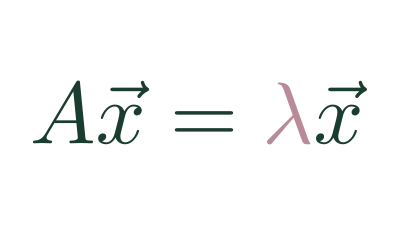A value which describes how much a transformation scales an Eigenvector

• ## Eigenvector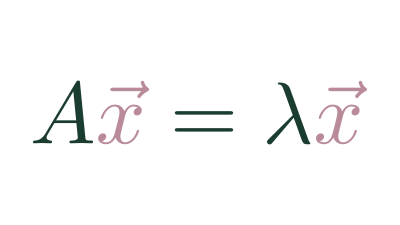A set of vectors whose span doesn't change after a transformation.

• ## Changing Basis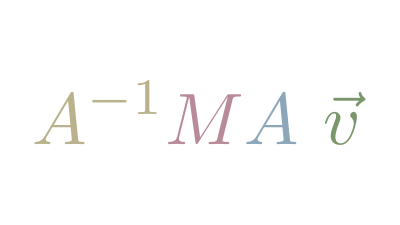Since any vectors can be Basis Vectors, it's useful to understand how to translate vectors between bases

• ## Cross Product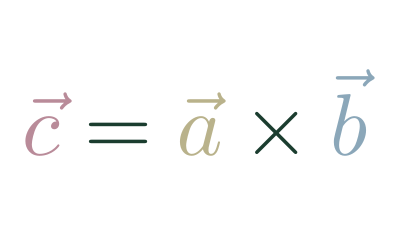An operation between two 3d vectors that returns a vector.

• ## Matrix Determinate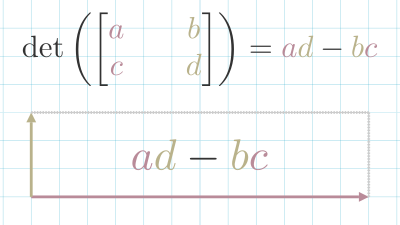A measure of how a matrix scales space.

• ## Basis Vectors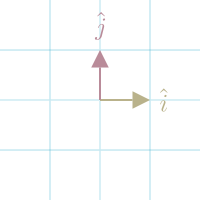The set of vectors that defines space.

• ## Identity Matrix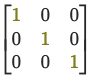When you multiply a matrix $(A)$ by the Identity Matrix $(I)$, you get the original matrix back.

$A …$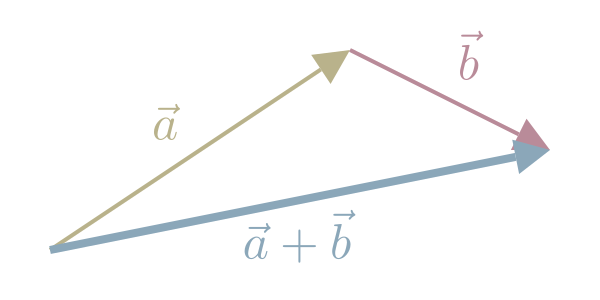$\stackrel{⃗}{a}+\stackrel{⃗}{b}=\left[\begin{array}{c}{a}_{1}\\ {a}_{2}\end{array}\right]+\left[\begin{array}{c}{b}_{1}\\ {b}_{2}\end{array}\right]=\left[\begin{array}{c}{a}_{1}+{b}_{1 \dots }\end{array}$

• ## Vector Subtraction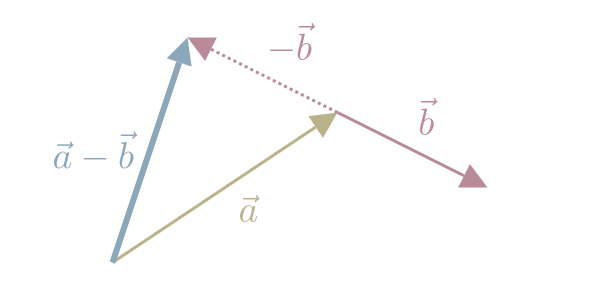We subtract one Vector from another by subtracting the corresponding components.

$\stackrel{⃗}{a}-\stackrel{⃗}{b}=\left[\begin{array}{c}{a}_{1}\\ {a}_{2}\end{array}\right]-\left[\begin{array}{c}{b}_{1}\\ {b}_{2}\end{array}\right]=\left[\begin{array}{c}{a}_{1}-{b}_{1 \dots }\end{array}$

• ## Vector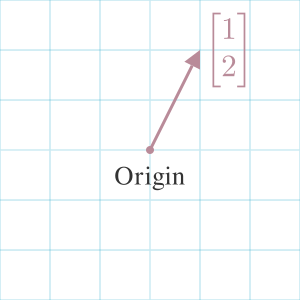An ordered list of numbers that describe an arrow in space.